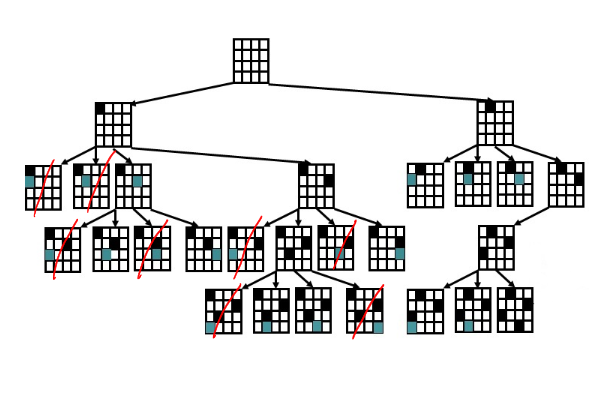# Constraint Satisfaction Problems in Artificial Intelligence

Original article was published on Artificial Intelligence on Medium# Constraint Satisfaction Problems in Artificial Intelligence

Constraint satisfaction problems are problems in Artificial Intelligence which concern themselves with strictly the goal state. They take advantage of general state representation.

## Intuition

States — represent them as a vector of feature values (a set of k variables), each variable has a domain of unique values. The state is specified by assigning values to all the variables, and a partial state is the assignment of a value to some of the variables

Goal — conditions on the vector of feature values

To solve a CSP we find a set of values for the features to satisfy the conditions.

A formal definition:

A CSP consists of

• A set of variables V1, …Vn;
• A(finite)domain of values Domain[Vi] ;
• A set of constraints C1, …, Cm

Each constraint has a scope or a set of variables that can be operated on.

The goal is to satisfy the constraint by assigning the right values. A CSP is cannot be satisfied if no solution exists.

A CSP problem can be defined as a search problem where the initial state is an empty assignment to a variable, the function is assigning values to variables and the goal test is to see if the assignments are correct(no constraint violations) and complete.

## Backtracking Search

Since CSPs do not require finding a path to a goal, they just require the correct configurations to the goal. We can solve these best by backtracking from the goal or reverse engineering the problem.

Method:

1. Find partial assignments
2. Decide on a value for one variable at a time (order agnostic)
3. If a constraint fails just reject it

Implementation:

You can complete backtracking recursively.

If the variables are set, then terminate the recursion

Else:

1. Pick an unassigned variable V and assign it a value

2. Test the constraints of V and all other variables that were assigned

2. If a constraint fails than return, else keep recursing.

## Constraint Propagation

There might be variables that have no possible values, but backtracking does not detect this until it tries to assign them a value.

We can look ahead at the unassigned variables trying to detect the failures.

Propagation has to be applied to the search and almost if not all every nodes in the tree. Since propagation is an inference, it takes a lot long to calculate the next step.

We can propagate using two ways:

Forward Checking and Generalized Arc Consistency.

## Forward Checking:

When instantiating a variable V, do the following for all constraints C that have only one not instantiated variable X remaining:

• Check all the values of X;
• Prune those values that violate C.

Then backtrack again.

As we backtrack, we have to restore the values that were pruned.